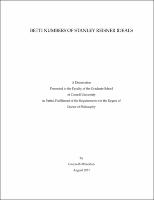## Betti Numbers Of Stanley-Reisner Ideals##### Abstract
This thesis compiles results in four related areas. • Jump Sequences of Edge Ideals: Given a graph G on n vertices with edge ideal IG , we introduce a new invariant Jump(IG ) which describes the possible Betti tables of IG . We show that the smallest k such that [beta]k,k+3 (IG ) = 0 is bounded below in terms of smallest j such that [beta]j,j +2 (IG ) = 0. In addition, we show that for ideals IG such that [beta]2,4 (IG ) = 0 and fewer than 11 vertices satisfy reg(IG ) [LESS-THAN OR EQUAL TO] 3. We construct large classes of examples partially spanning the set of Betti tables of IG with reg(IG ) = k . • Stabilization of Betti Tables: Let R be a polynomial ring. Given a homogeneous ideal I ⊆ R equigenerated in degree r, we show that the Betti tables of I d stabilize into a fixed shape for all d [GREATER-THAN OR EQUAL TO] D for some D. • Linear Quotients Ordering of Anticycle: Let An be the anticycle graph on n vertices and Pn be the antipath graph on n vertices. We produce a linear quotients ordering on all powers of the edge ideal of the antipath k IPn , and a linear quotients order on the second power of the edge ideal of 2 the anticycle anticycle IAn . • Nerve Complexes of Graphs: We examine the nerve complex N (G) of a graph G. We show that the Betti numbers of this complex encode spanning trees, matchings, genus, k -edge connectivity, and other invariants of G.
2011-08-31
##### Keywords
Commutative Algebra; Betti Numbers; Free Resolutions and Syzygies
##### Committee Chair
Stillman, Michael Eugene
##### Committee Member
Peeva, Irena Vassileva
Swartz, Edward B.
Mathematics
##### Degree Name
Ph. D., Mathematics
##### Degree Level
Doctor of Philosophy
##### Types
dissertation or thesis